© SQL :: Manager Home Program Information Technical details Free download Donate for a cup of coffee
 Installation & program start Program structure System administrationUser settingsMySQL ServerMySQL DatabasesMySQL TablesMySQL PartitionsSystem-versioned tablesMariaDB SequencesMySQL ViewsMySQL ProceduresMySQL FunctionsMariaDB PackagesMySQL TriggersMySQL EventsSQL statements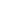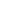# The CONV SQL Function in MySQL and MariaDB - Convert numbers

 CONV Syntax: CONV(Value, from Base, to Base) Return value: CHAR Function type: Conversion function
 Function Description

The CONV() function converts numbers between different number systems.

CONV() returns a string of "Value" converted "from Base" "to Base".

If "Value" is NULL, or if "from Base" or "to Base" are invalid, CONV() returns NULL back.

The minimum base is 2 and the maximum base is 36.

Similar fixed base functions are: BIN(), OCT(), HEX() and UNHEX().

The "Value" argument is interpreted as an integer, but can also be specified as a character string.

If "Value" is not an integer, 0 is returned.

If "to Base" is a negative number, "Value" is considered a signed number, otherwise unsigned.

CONV() works with 64-bit precision.

Specifying hexadecimal and binary literal values in argument ​​only works to a limited extent in MariaDB:

## Examples running on MariaDB 10.5

conv(10, 10, 16)
varchar(64) BINARY
A
conv(10, 10, 8)
varchar(64) BINARY
12
conv(10, 10, 4)
varchar(64) BINARY
22
conv(10, 10, 2)
varchar(64) BINARY
1010
conv('10', 10, 2)
varchar(64) BINARY
1010
conv(-10, 10, 8)
varchar(64) BINARY
1777777777777777777766
conv(-10, 10, -8)
varchar(64) BINARY
-12
conv('ff', 16, 10)
varchar(64) BINARY
255
conv('ff00', 16, 10)
varchar(64) BINARY
65280
conv('1111', 2, 10)
varchar(64) BINARY
15
conv('1111', 2, 16)
varchar(64) BINARY
F
conv(0xff00, 10, 10)
varchar(64) BINARY
0
conv(0xff00 + 0, 10, 10)
varchar(64) BINARY
65280
conv(0b1111, 10, 10)
varchar(64) BINARY
0
conv(0b1111 + 0, 10, 10)
varchar(64) BINARY
15
conv('test', 10, 2)
varchar(64) BINARY
0
conv(null, 10, 2)
varchar(64) BINARY
NULL
conv(10, 10, 1)
varchar(64) BINARY
NULL

## Examples running on MySQL 8.0.23

conv(10, 10, 16)
varchar(65) BINARY
A
conv(10, 10, 8)
varchar(65) BINARY
12
conv(10, 10, 4)
varchar(65) BINARY
22
conv(10, 10, 2)
varchar(65) BINARY
1010
conv('10', 10, 2)
varchar(65) BINARY
1010
conv(-10, 10, 8)
varchar(65) BINARY
1777777777777777777766
conv(-10, 10, -8)
varchar(65) BINARY
-12
conv('ff', 16, 10)
varchar(65) BINARY
255
conv('ff00', 16, 10)
varchar(65) BINARY
65280
conv('1111', 2, 10)
varchar(65) BINARY
15
conv('1111', 2, 16)
varchar(65) BINARY
F
conv(0xff00, 10, 10)
varchar(65) BINARY
65280
conv(0xff00 + 0, 10, 10)
varchar(65) BINARY
65280
conv(0b1111, 10, 10)
varchar(65) BINARY
15
conv(0b1111 + 0, 10, 10)
varchar(65) BINARY
15
conv('test', 10, 2)
varchar(65) BINARY
0
conv(null, 10, 2)
varchar(65) BINARY
NULL
conv(10, 10, 1)
varchar(65) BINARY
NULL
 Updated: 2023-09-24 Imprint Contact Terms & conditions Privacy & Cookies Updates Sitemap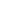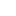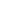© 2020-2023 Heino Cunze-Fischer